### 3.4. The Kompaneets approximation

The calculations that led to equation (51) are accurate to the appropriate order in photon frequency and electron energy for our purposes, and take account of the relativistic kinematics and statistics of the scattering process. In the non-relativistic limit the scattering process simplifies substantially, and may be described by the Kompaneets (1956) equation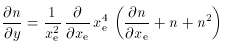(52)

which describes the change in the occupation number, n() by a diffusion process. In (52), xe = h/ kB Te, which should not be confused with x = h/ kB Trad used previously, and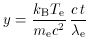(53)

is a dimensionless measure of time spent in the electron distribution. (4)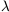e is the ``Compton range'', or the scattering mean free path, (neT)-1. For a radiation field passing though an electron cloud, y, which is usually known as the Comptonization parameter, can be rewritten in the more usual form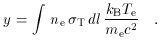(54)

Note that a time- (or y-) independent solution of (52) is given when the electrons and photons are in thermal equilibrium, so that n = (exe -1 )-1, as expected, and that the more general Bose-Einstein distributions n = (exe+-1)-1 are also solutions. A derivation of equation (52) from the Boltzmann equation is given by Bernstein & Dodelson (1990).

In the limit of small xe, which is certainly appropriate for the CMBR and hot electrons, ð n / ð xe >> n, n2, and (52) becomes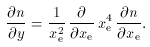(55)

The homogeneity of the right hand side of this equation allows us to replace xe by x, and a change of variables from x, y to, y, where= 3y + ln xe, reduces (55) to the canonical form of the diffusion equation,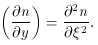(56)

In the format of equation (49), this indicates that the solution of the Kompaneets equation can be written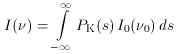(57)

where the Kompaneets scattering kernel is of Gaussian form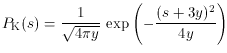(58)

(Sunyaev 1980; Bernstein & Dodelson 1990). The difference between the scattering kernels (33) and (58) is small but significant for the mildly relativistic electrons that are important in most cases, and can become large where the electron distribution becomes more relativistic (compare the solid and dashed lines in Fig. 5). These shape changes lead to spectral differences in the predictedI (), which for thermal electrons can be characterized by the changing positions of the minimum, zero, and maximum of the spectrum of the Sunyaev-Zel'dovich effect with changing electron temperature. This is illustrated in Fig. 9 for a range of temperatures that is of most interest for the clusters of galaxies (Section 4).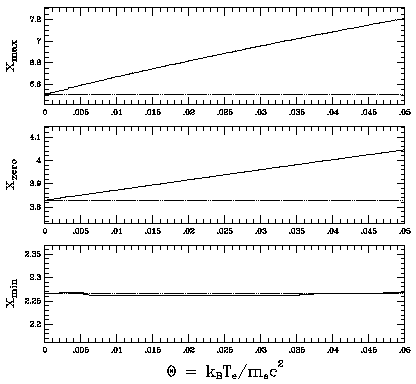Figure 9. The variation of the positions of the minimum, zero, and maximum of the spectrum of the thermal Sunyaev-Zel'dovich effect,I (x), as the electron temperature varies. The positions of the spectral features are described by the dimensionless frequency x = h/ kB Trad, and the electron temperature is characterized by= kB Te / me c2.

Most work on the Sunyaev-Zel'dovich effect has used the Kompaneets equation, and implicitly the Kompaneets scattering kernel, rather than the more precise relativistic kernel (44) advocated by Rephaeli (1995a). The two kernels are the same at small Te, as expected since the Kompaneets equation is correct in the low-energy limit. At low optical depth and low temperatures, where the Comptonization parameter y is small, and for an incident photon spectrum of the form of equation (15), the approximation ð n / ð y =n / y may be used in (55) to obtain a simple form for the spectral change caused by scattering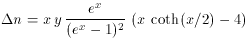(59)

with a correspondingI (x) = x3n (x) I0, where I0 = 2 h / c2 [(kB Trad / h)]3 again. This result can also be obtained directly from the integral (57) for I (x) in the limit of small y. Fig. 7 compares the Kompaneets approximation forI (x) with the full relativistic results.

There are three principal simplifications gained by using (59), rather than the relativistic results.

 1 The spectrum of the effect is given by a simple analytical function (59). 2 The location of the spectral maxima, minima, and zeros are independent of Te in the Kompaneets approximation, but vary with Te in the relativistic expressions. To first order in, which is adequate for temperatures kB Te < 20 keV (< 0.04), xmin = 2.26 xzero = 3.83(1 + 1.13) (60) xmax = 6.61(1 + 2.15) as seen in Fig. 9. Earlier approximations for xzero() are given by Fabbri (1981) and Rephaeli (1995a). 3 The amplitude of the intensity (or brightness temperature) change depends only on y (Tee) in the Kompaneets approximation, but in the relativistic expression the amplitude is proportional toe (for smalle) and also depends on a complicated function of Te.

It is possible to improve on the Kompaneets result (59) by working to higher order infrom (51) or the Boltzmann equation. The resulting expressions forn orI are usually written as a series in increasing powers of(Challinor & Lasenby 1998; Itoh et al. 1998; Stebbins 1998). Taken to four or five terms these series provide a useful analytical expression for the Sunyaev-Zel'dovich effect for hot clusters for a wide range of x. However, the expressions cannot be used blindly since they are asymptotic approximations, and are still of poor accuracy for some (x,) values. The approximations also rely on the assumptions that the cluster is optically thin and that the electron distribution function is that of a single-temperature gas (35). Both assumptions are questionable when precise results (to better than 1 per cent) are needed, and so the utility of the idealized multi-term expressions is limited. For the most precise work, especially work on the kinematic Sunyaev-Zel'dovich effect (Section 6), the relativistic expressions with suitable forms for the distribution functions, and proper treatment of the cluster optical depth, must be used if accurate spectra for the effect, and hence estimates for the cluster velocities, are to be obtained (Molnar & Birkinshaw 1998b).

4 (53) corrects equation (11) in the Kompaneets paper for an obvious typographical error. Back.